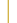Introduction Free SoftCDXASCHOOCHDARESBURYEDAEXAFS (pour le Mac)EXAFSPAKGNXASLASEMURATANPISEDEMTT-MULTIPLETSVIPERXAFSXAIDXANES dactyloscope Commercial SoftCerius2 XAFSExcurv98XDAP Licensed SoftFEFFUWXAFSWINXASXFIT Links [TOP]
 VIPER (Visual Processing in EXAFS Researches) HARDWARE Windows DOWNLOAD Download the latest version here AUTHORS K.V.Klementev NOTES Several glitch corrections on absorptance curve with simultaneous observation of the EXAFS curve. Before this, glitches are detected on the derivative level of the first ionization chamber current. Deconvolution of absorption coefficient with monochromator resolution curve. Given XPS-spectrum (measured or calculated) near and deeper than the chosen absorption edge, one can extract a one-electron absorption from the total one (by means of Bayesian deconvolution procedure) and use then a quite clear one-electron theory without complicated many-body corrections. An EXAFS-part, chi(k), extraction. Three methods for construction of the atomic-like absorption are realized: (i) by a spline drawn through the knots varied to minimize low-r EXAFS part; (ii) by a smoothing spline and (iii) by a Bayesian smoothing curve. The last two can take into account a priori information about atomic-like absorption (edge shape, AXAFS, multi-electron contribution, etc.). chi(k) extraction can be verified simultaneously by Fourier-transform and back Fourier-transform. chi(k) or chi(E) can be corrected by arbitrary functions f(k) or f(E). Errors of chi(k) are calculated by various ways in order to validate the spectrum extension. Fast Fourier analysis, k-weighting, several windowing functions. Phase and amplitude corrections. Decomposition into amplitude and phase and subsequent calculation of the EXAFS amplitude and phase. Fitting procedures for the first few coordination shells not influenced by the multiple-scattering contributions: By ordinary EXAFS formula. Using the oscillatory potential U(r) of the absorber-scatterer pair. The potential U(r) is set by typed in arbitrary formula, parameters of which then are varied. U(r) may consist of many wells. Both classical and quantum approaches are used. All fit parameters can be fixed and constrained or related to the homogeneous parameters of the other spheres (wells) by arbitrary functions. Fitting is done either in k- or in r-space. Scattering amplitudes and phases must be calculated by other programs (FEFF format is recognized). The parameters and results of fitting can be saved in (and then a model can be loaded from) a database. Advanced error analysis is developed and applied. Statistical chi^2- and F- tests can be performed at user request. Any time, all curves and their changes under processing are visual. Several parameters can be quickly changed by mouse dragging. Data file structure is tunable and flexible, no previous conversion needed to load multiple column files from different EXAFS stations. [TOP]